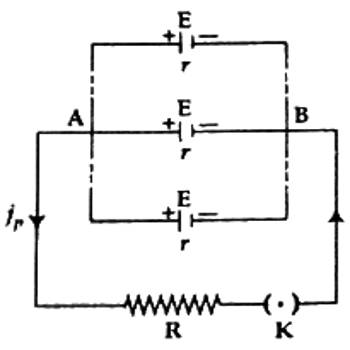Physics

# Parallel Combination of a CellParallel combination: If the positive plates of some electric cells are connected at one point and negative plates are connected at another point, then the combination of the cells is called a parallel combination. A cell is an electrical element which produces current and a battery is a set of some cells with series or parallel connection.Let a resistor of value R be connected to ‘n’ number of electric cells in parallel combination. Electromotive force and internal resistance of each cell are respectively E and r. Since the positive plates of the cells are connected at one point A and negative plates at another point B, so the electromotive force of the battery or combination will be equal to the electromotive force of any one cell. Again, since cells are connected in the parallel combination, so their internal resistances are connected in parallel. Hence if the internal resistance of the combination of cells or battery is RP, then,

1/RP = 1/r + 1/r + … … … n number = n/r.

So, RP = r/n

So, total resistance of the circuit = R + RP = R + r/n. If current flowing through the cell is iP, then awarding to Ohm’s law,

iP = [total electromotive force / total resistance]

= E / (R + r/n) = nE/(nR + r)

E nE

r srR + r R +

(1) If nR is very large compared to r, then r can be ignored, so we get,

iP = nE/nR = = E/R = current produced by a single cell.

In this situation, the elf efficiency of the battery does not increase.

(2) If nR is very small compared to r, then, iP = nE/r = n x current produced by a single cell.

In this situation, the efficiency of the battery increases enormously.